## Example Questions

### Example Question #1 : Percentage

Column A: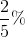Column B: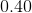The two quantities are equal.

The relationship cannot be determined from the given information.

Column A is greater.

Column B is greater.

Column B is greater.

Explanation:

2/5% = 0.40% = 0.004.  Therefore, Column B is greater.

### Example Question #7 : Percentageispercent ofispercent of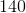Quantity A:Quantity B:The relationship cannot be determined.

Quantity B is greater.

Quantity A is greater.

The two quantities are equal.

Quantity B is greater.

Explanation:

To do this problem, translate each expression into mathematical terms:ispercent of: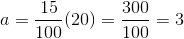ispercent of: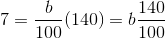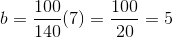Quantity B is greater.

### Example Question #8 : Percentage

Quantity A: 20% ofQuantity B: 30% of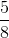Quantity A is greater.

The two quantities are equal.

The relationship cannot be determined.

Quantity B is greater.

Quantity B is greater.

Explanation:

To solve this problem, consider writing the quantities in terms of only fractions:

Quantity A: 20% of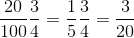Quantity B: 30% of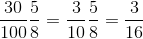Quantity B has the smaller denominator for an equal numerator. There is no need for calculation beyond this, and time on the GRE would be best spent elsewhere.

Quantity B is greater.

Tired of practice problems?

Try live online GRE prep today.